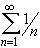#Interactive Real Analysis

Next | Previous | Glossary | Map

## 4.3. Special Series

### Definition: Harmonic Series

The series= 1 + 1/2 + 1/3 + 1/4 + 1/5 + ... is called harmonic series. It diverges to infinity.

For an interesting application of the harmonic series, check the story about the Leaning Tower of Lire.

### Proof:

We need to estimate the n-th term in the sequence of partial sums.

### You browser is not 'Java-enabled' ...

The n-th partial sum for this series is:
S N = 1 + 1/2 + 1/3 + 1/4 + ... + 1/n
Now consider the following subsequence extracted from the sequence of partial sums:
S 1 = 1
S 2 = 1 + 1/2
S 4 = 1 + 1/2 + (1/3 + 1/4)1 + 1/2 + (1/4 + 1/4) = 1 + 1/2 + 1/2 = 1 + 2/2
S 8 = 1 + 1/2 + (1/3 + 1/4) + (1/5 + 1/6 + 1/7 + 1/8)1 + 1/2 + (1/4 + 1/4) + (1/8 + 1/8 + 1/8 + 1/8) = 1 + 1/2 + 1/2 + 1/2 = 1 + 3/2
In general, one can use induction (do it as an exercise) to show that
S 2 k1 + k / 2
for all k. Hence, the subsequence { S 2 k } extracted from the sequence of partial sums { S N } is unbounded. But then the sequence { S N } can not converge either and must diverge to infinity.Next | Previous | Glossary | Map